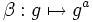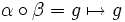# Kth power map is bijective iff k is relatively prime to the order

## Statement

Suppose$G$ is a finite group and$k$ is a natural number. Then, the map$g \mapsto g^k$ is bijective from$G$ to$G$ if and only if$k$ is relatively prime to the order of$G$.

Note that the power map (also termed a universal power map) is not necessarily an endomorphism of$G$. If it is also an endomorphism, then its being bijective is equivalent to its being an automorphism.

## Facts used

1. Order of element divides order of group, or equivalently, Exponent divides order: For any finite group$G$ and any element$g \in G$, we have that the order of$g$ divides the order of$G$. In particular,$g^{|G|}$ is the identity element. Equivalently, the exponent of$G$, which is defined as the least common multiple of the orders of all its elements, divides the order of$G$.
2. Cauchy's theorem: If$p$ is a prime divisor of the order of$G$, then$G$ contains an element of order$p$. Equivalently, exponent of a finite group has precisely the same factors as order.

## Proof

### Relatively prime to the order implies power map is bijective

Given: A finite group$G$, a natural number$k$ relatively prime to the order of$G$.

To prove: The map$\alpha: g \mapsto g^k$ is a bijection from$G$ to itself.

Proof: Let$n$ be the order of$G$. Since$k$ and$n$ are relatively prime, the subgroup of$\mathbb{Z}$ generated by$k$ and$n$ is the whole group$\mathbb{Z}$. In particular, we can find integers$a,b \in \mathbb{Z}$ such that$ak + bn = 1$.

Consider the map$\beta:g \mapsto g^a$. We now have:$\alpha \circ \beta = g \mapsto (g^k)^a = g^{ka} = g^{1 - bn} = g.(g^n)^{-b}$.

By fact (1),$g^n$ is the identity element, so we get:$\alpha \circ \beta = g \mapsto g$

which is the identity map on$G$. Similarly,$\beta \circ \alpha$ is the identity map on$G$. Thus, both$\alpha$ and$\beta$ are bijective maps, completing the proof.

### Power map is bijective implies relatively prime to the order

Given: A finite group$G$, a natural number$k$ that is not relatively prime to the order of$G$.

To prove: The map$\alpha:g \mapsto g^k$ is not a bijection.

Proof: Since$k$ and$n$ are not relatively prime, there exists a common prime divisor$p$ of both$k$ and$n$. By fact (2), there exists a non-identity element$g$ such that$g^p$ is the identity element. Thus, we get that$g^k = e$, where$e$ is the identity element. Thus,$\alpha(g) = g^k = e = e^k = \alpha(e)$, contradicting injectivity.